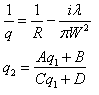BRIGHAM YOUNG UNIVERSITY
 Search BYUContact   |   Help[expand all...]
[COLLAPSE ALL...]# Gaussian Beam Propagation Calculator:

Enter in the following parameters about the Gaussian beam. You can use scientific notation (e.g. 1550 nm = 1550e-9).
 Wavelength (λ): [m] Beam Radius (at Ioe-2): [m]

Enter the following parameters about your lenses. Enter a negative focal length for convex (beam expanding) lenses. Leave fields blank if a lens is not used.
1. Typical focal lengths for microscope objectives

Distance to Lens: Focal Length of Lens:
For lens 1 [m] [m]
For lens 2 [m] [m]
For lens 3 [m] [m]
For lens 4 [m] [m]

Enter in the final propagating distance
 Final Propagation Distance (in Meters): [m]

The following are calculated values of the system
At lens 1 [m] [m]
At lens 2 [m] [m]
At lens 3 [m] [m]
At lens 4 [m] [m]
At End of System [m] [m]

## Equations used above:These equations are used to calculate the initial radius of curvature (R) and the initial beam radius (W).In the equations above R is the radius of curvature, W is the beam radius, i is the square root of negative 1 and &lambda is the wavelength.
For a Gaussian beam propagating through a lens A=1, B=0, C=-1/f (where f is the focal length) and D=1.
For a Gaussian beam propagating through air A=1, B=d (where d is the distance), C=0 and D=1.Maintained by ECEn IMMERSE Web Team.